# How to Calculate Age in Excel from Birthday?

Suppose you have compiled a list of the student’s dates of birth in an Excel spreadsheet, and at this point, you need to figure out how old each one of them is. Excel has a number of useful functions, like DATEDIF and YEARFRAC, that can make it easy to figure out a student’s age based on their birth date.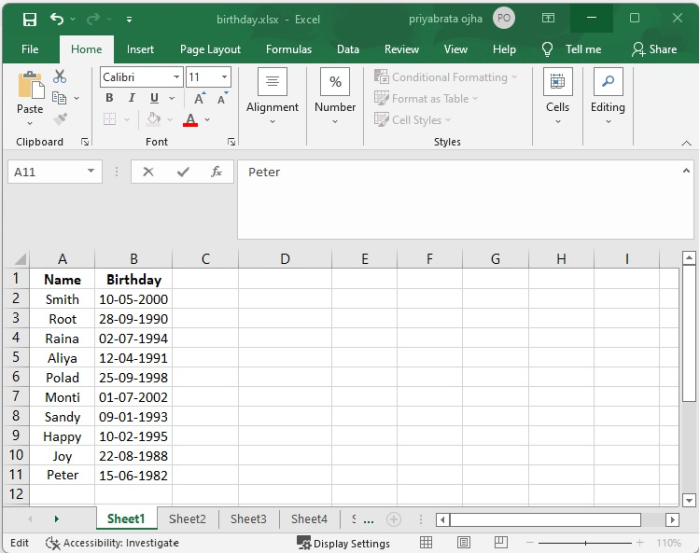## Using the DATEDIF Function

The DATEDIF function can convert the birth date to the current age. The general syntax for this is as follows −

=DATEDIF(birthdate,TODAY(),"y")

Where,

• Birthdate − The date that corresponds to the student's actual birthday.

• TODAY() − The current date is what is returned by this function.

• y − It provides the total number of years that have passed from the birth date, based on the current date to the birth date.

Step 1 − The ages of everyone have been determined based on the birth date, as seen in the following screenshot −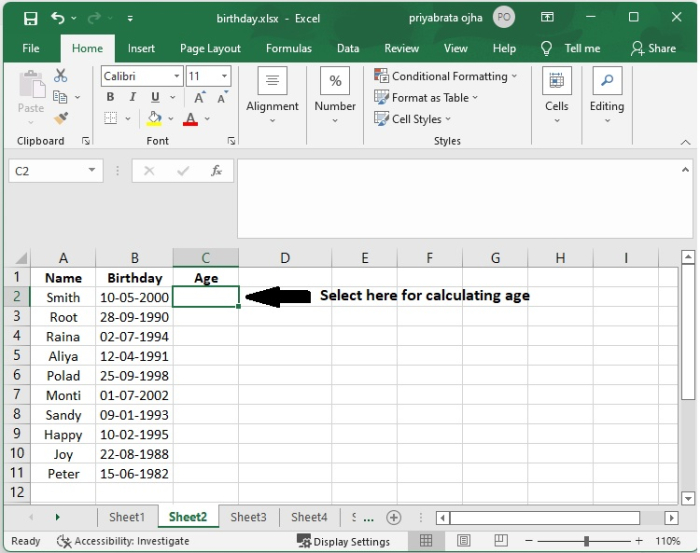Step 2 − The formula that is used to determine a student’s age is as follows −

=DATEDIF(B2,TODAY(),"y")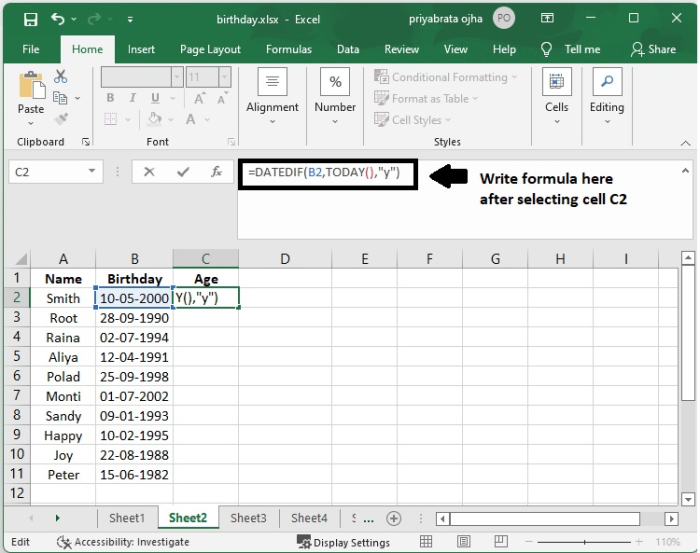Step 3 − The result of the calculation to determine Smith's age in C2 cells is shown below.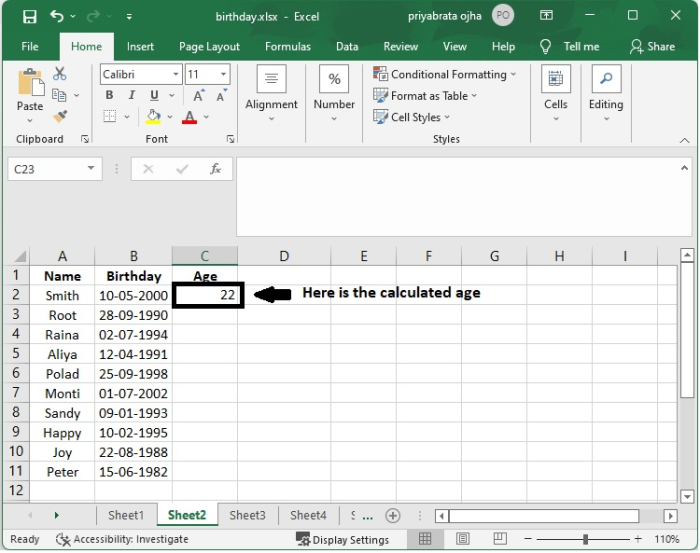Step 4 − After that, just drag the fill handle down to the cells in which you wish to apply this formula. At this point, all of the ages would have been computed and presented as decimal values in the cells.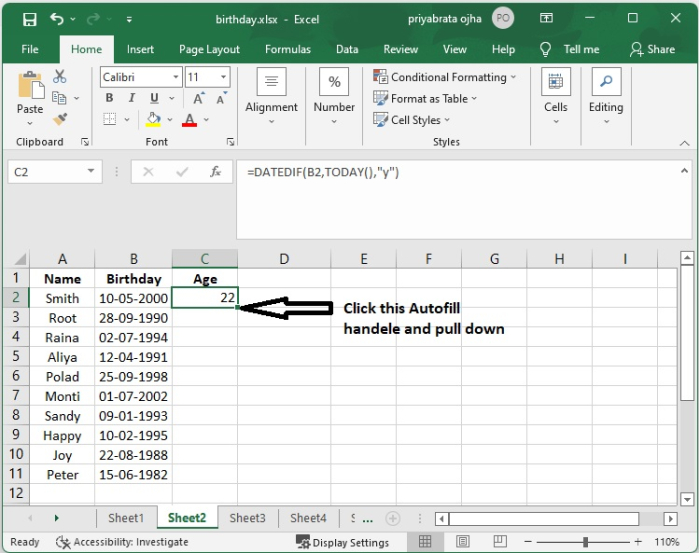Step 5 − Now we can get the current age‘s of all the students from their date of birth as shown in the following screenshot.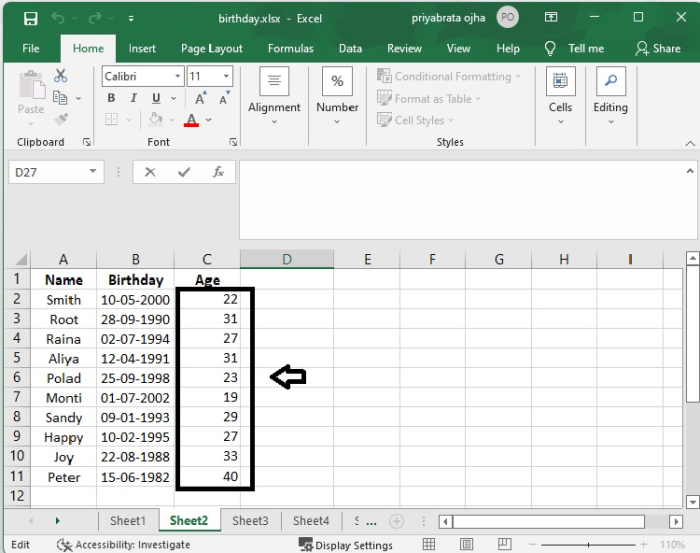## Using the YEARFRAC Function

The YEARFRAC function will assist you in calculating your age based on the birth date that you provide; the general syntax for this function is as follows −

=YEARFRAC(birthdate,TODAY())

Where,

• Birthdate − The date that corresponds to the student's actual birthday.

• TODAY() − The current date is what is returned by this function.

Step 1 − In addition, the ages of everyone have been determined based on the birth date, as seen in the screenshot −Step 2 − If you want the Age number to be an integer, you should combine the YEARFRAC function with the INT function in the following manner. The computed result will be a decimal number if you use the YEARFRAC function.

=INT(YEARFRAC(B2,TODAY()))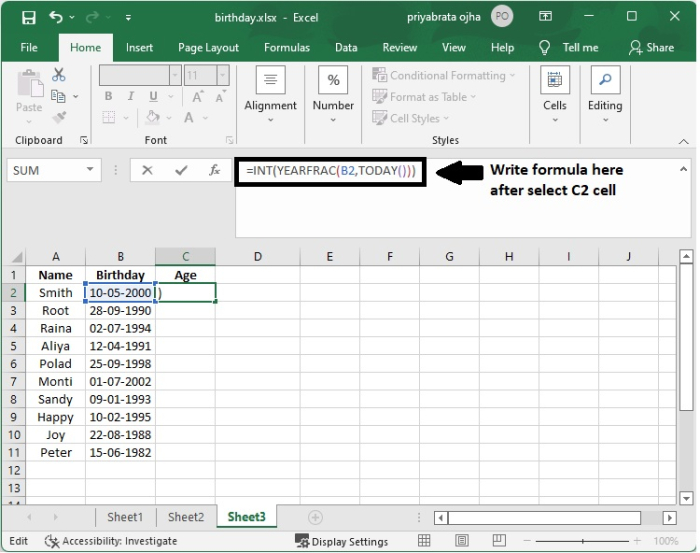Step 3 − The result of the calculation to determine Smith's age in C2 cells is shown below.Step 4 − After that, just drag the fill handle down to the cells in which you wish to apply this formula. At this point, all of the ages will have been computed and presented as decimal values in the cells; for example, check the screenshot.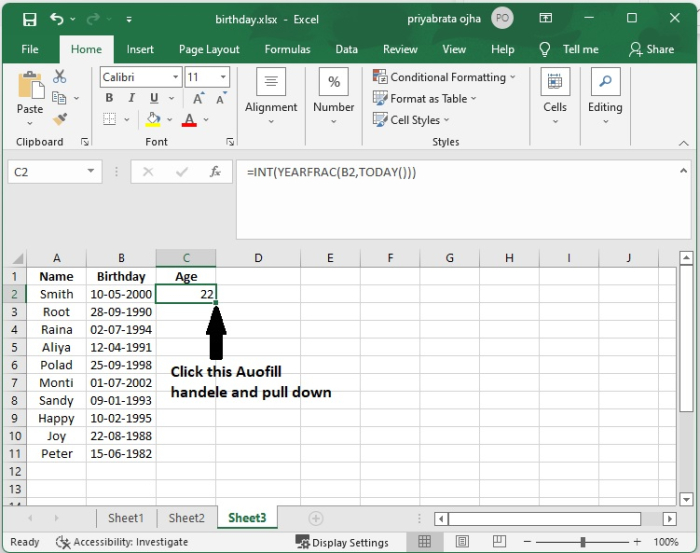Step 5 − Now we can get the current ages of all the students from their date of birth as shown in the following screenshot.## Conclusion

In this tutorial, you learnt how to use the DATEDIF and YEARFRAC methods in Excel to calculate the age of a person from his/her Birthday.